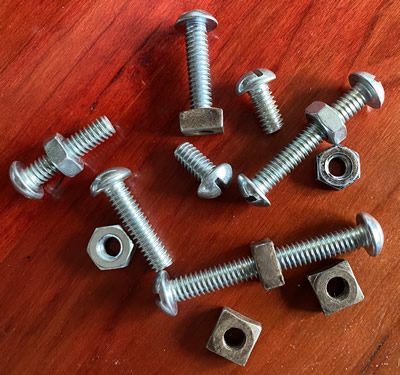# Activity lab notes to model Atoms, Elements, and CompoundsConcepts:

• Nuts and Bolts can be used to make a model of compounds.
• Compounds are chemically bonded elements.

Activity 1:

Pretend the nuts and bolts are different kinds of atoms.

Get the following from the instructor.

1 long bolt    1 short bolt

2 hex nuts     2 square nuts

Place both kinds of nut atoms and bolt atoms in separate piles on your desk.

Short bolt        Hex nut         Square nut         Long bolt

How many different kinds of atoms do these nuts and bolts represent?

If each kind of atom makes up a different element. How many different elements are represented?

In order to make a compound, at least two atoms have to combine or be bonded together.  For example, a short bolt atom may be combined (bonded) to a square nut atom.

A sketch representing this combination could be:

Assuming that SB is a symbol for a short bolt atom and SN a symbol for a square nut atom, the above combination could be described by the following symbol SBSN.

What would the following symbols represent? SBSN2

What does the subscript 2 mean?

Make a sketch representing this combination.

The symbol for a long bolt atom and a hex nut atom are shown below.

LB                       HX

If a long bolt atom and hex nut atom were combined

What would the shorthand symbol be?

• Use 1 long bolt, 1 short bolt, one square nut and one hex nut to determine how many other different combinations (compounds) can be made.
• Once a combination is made (1) draw it and (2) write its symbol in Data Table #1.
• Then take the combination apart and try again.
• Those already constructed have been entered in the table.
• Darken the heads of long bolts in your sketch.  This will serve to distinguish them from short ones.

Data Table #1

 Picture of Combination Shorthand Symbol BoSq B1HX

How many different compounds did you make?

To be counted as a different compound the combination has to have a different formula.

How do your combinations compare to another teams?

The set off symbols in the table above are also called formulas

A symbol represents one kind of atom, but, a formula represents a combination of more than one kind of atom.  It tells the number and kinds of bolts and nuts (atoms) held (bonded) together.

What is the formula for the combination of two short bolts and two square nuts?

What do the subscripts mean?

Using long bolts and hex nuts, make a compound that has the formula LB2HX2 and sketch it.

Could it be made in a different way?  If so, sketch it.

What information about a combination is lost by just using the symbol?

Sketch the number of different ways two long bolts can be combined with four square nuts.  All four nuts have to be used each time.

Data Table #2

 Picture of Combination Formula

Shorthand symbols could be used to describe how to form a combination (compound).  For example, a combination of two long bolts and two hex nuts are threaded together.

This could be described in words

A ( _______________________) is made from

TWO LONG BOLTS ( ______________)     PLUS TWO HEX NUTS     ( ______________)

TWO-BOLT-TWO-NUT    COMBINATION (______________)

or pictures

or by using symbols

2LB + 2Hx  ->   LB2Hx2

What does the LB and Hx to the left of the arrow represent?

What does the arrow mean?

What does the formula, LB2Hx2 to the right of the arrow mean?

What do the prefix numbers represent on the left side of arrow?

What about the subscript numbers to the right of the arrow?

Create your own interaction with bolts and nuts:

Interaction 1

Draw a Diagram

Write a word description of this interaction.

Now, write a description using symbols.

Interaction 2

Draw a Diagram

Write a word description of this interaction.

Now, write a description using symbols.

There are millions of different substances in the universe.  However, scientists believe that everything on the earth and the stars are made of various combinations of only about 100 basic substances called elements.

Some Elements and symbols

 Name Symbol Name Symbol Name Symbol Aluminum Al Iodine I Oxygen O Calcium Ca Iron Fe Potassium K Carbon C Lead Pb Silver Ag Chlorine CI Magnesium Mg Sodium Na Copper Cu Mercury Hg Sulfur S Helium He Nitrogen N Zinc Zn Hydrogen H

The penny is made of copper.  What is its symbol?

Many beverage cans are made of aluminum.  What is its symbol?

The oxygen found in air is made of two atoms.  Write its formula.

The nitrogen in air is also made of two atoms.  What would its formula be?

When atoms of elements combine, compounds are formed.  A compound is identified by two things:

1. the kinds of atoms in it and
2. the number of each kind of atom.

The formula for the compound water is H2O.

What atoms are found in water?

How many of each kind of atom are there?

Complete the table below by adding the missing information.

 Substance Formula kind of atom Number of each Atoms Water H2O H 2 O 1 Table Salt NaC1 Na Cl Dry Cleaning Fluid CC14 Sugar C12H22O11 Ammonia NH3 Baking Soda NaHCO3 Rust FeO